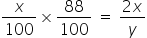# CAT-2021-QA-Slot-1/Free CAT Question papers

6).

Identical chocolate pieces are sold in boxes of two sizes, small and large. The large box is sold for twice the price of the small box. If the selling price per gram of chocolate in the large box is 12% less than that in the small box, then the percentage by which the weight of chocolate in the large box exceeds that in the small box is nearest to

1. 127

2. 135

3. 124

4. 144

### Explanation

Let the small box and the large box are sold at x and 2x rupees respectively. Also, let weight of chocolate in small box and large box respectively is 100 gram and y gram respectively. Then

price per gram in small box = x/100 and

price per gram in large box = 2x/y

As per the question,

== > y = 20000/88 = 2500/11 = 227

which is about 127 % more than weight of small box.

Ans (1)